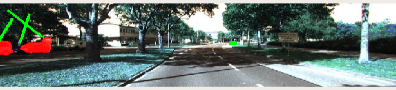# How to create Axis-aligned bounding boxes using cv2.Flatten()

I am trying to get axis-aligned bounding box where the original algorithm used rotated bounding boxes. corresponding lines are –
if len(contours) != 0 and np.max(cnt_area) > 100:
contour = contours[np.argmax(cnt_area)] # use max area polygon
polygon = contour.reshape(-1, 2)
pbox = cv2.boundingRect(polygon) # Min Max Rectangle
#pbox = cv2.boxPoints(cv2.minAreaRect(polygon))
box_in_img = pbox
state[‘ploygon’] = rbox_in_img if mask_enable else []
location = state[‘ploygon’].flatten()

But getting the following error: tuple object doesnot have flatten. but flatten() previously worked with rotated bounding boxes.
Then if I delete the flatten() function, I do not get errors but bounding boxes are not correct.Can someone give suggestions to solve this?